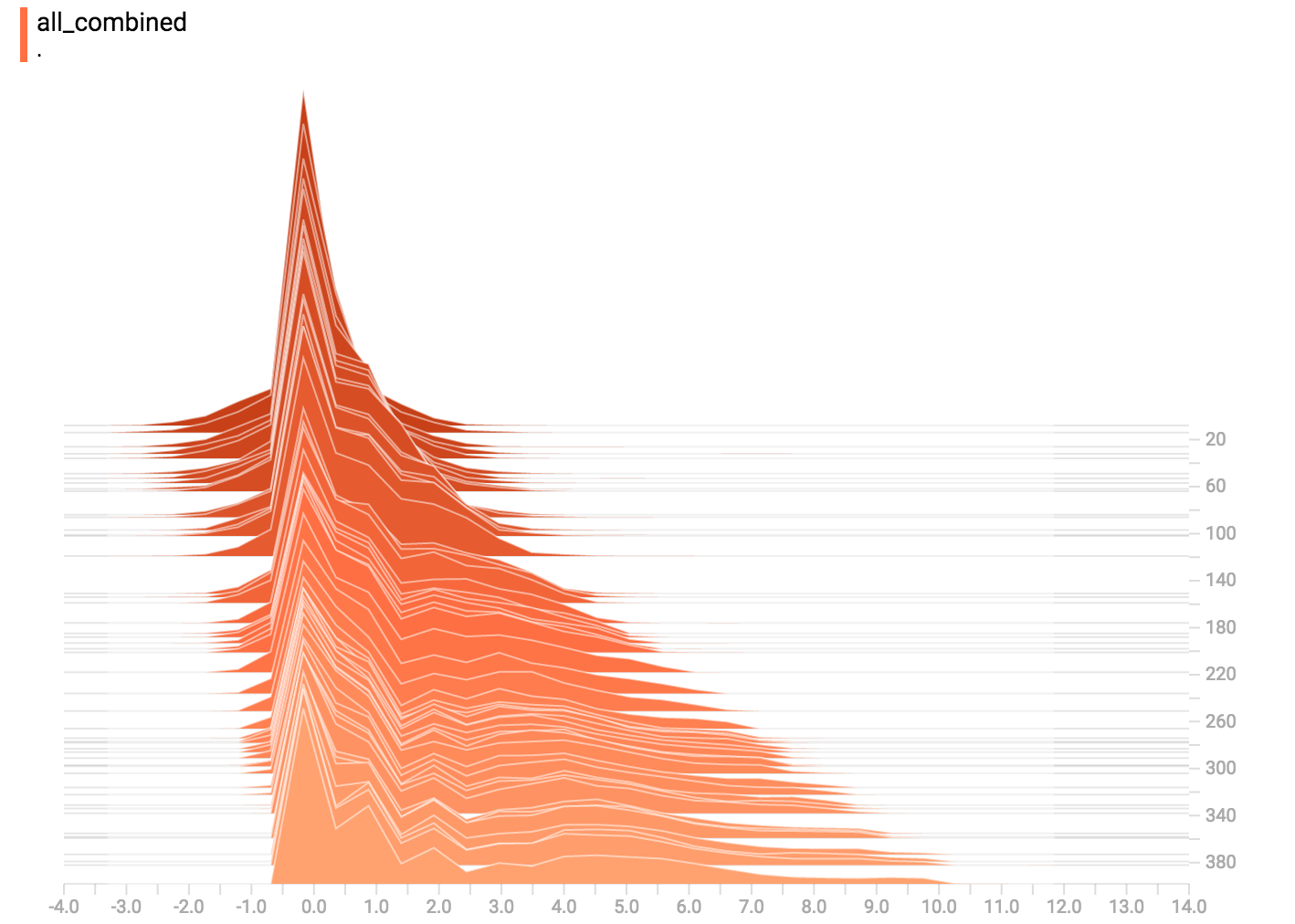Missed TensorFlow World? Check out the recap.

# TensorBoard Histogram Dashboard

The TensorBoard Histogram Dashboard displays how the distribution of some `Tensor` in your TensorFlow graph has changed over time. It does this by showing many histograms visualizations of your tensor at different points in time.

## A Basic Example

Let's start with a simple case: a normally-distributed variable, where the mean shifts over time. TensorFlow has an op `tf.random_normal` which is perfect for this purpose. As is usually the case with TensorBoard, we will ingest data using a summary op; in this case, 'tf.summary.histogram'. For a primer on how summaries work, please see the TensorBoard guide.

Here is a code snippet that will generate some histogram summaries containing normally distributed data, where the mean of the distribution increases over time.

``````import tensorflow as tf

k = tf.placeholder(tf.float32)

# Make a normal distribution, with a shifting mean
mean_moving_normal = tf.random_normal(shape=, mean=(5*k), stddev=1)
# Record that distribution into a histogram summary
tf.summary.histogram("normal/moving_mean", mean_moving_normal)

# Setup a session and summary writer
sess = tf.Session()
writer = tf.summary.FileWriter("/tmp/histogram_example")

summaries = tf.summary.merge_all()

# Setup a loop and write the summaries to disk
N = 400
for step in range(N):
k_val = step/float(N)
summ = sess.run(summaries, feed_dict={k: k_val})
``````

Once that code runs, we can load the data into TensorBoard via the command line:

``````tensorboard --logdir=/tmp/histogram_example
``````

Once TensorBoard is running, load it in Chrome or Firefox and navigate to the Histogram Dashboard. Then we can see a histogram visualization for our normally distributed data.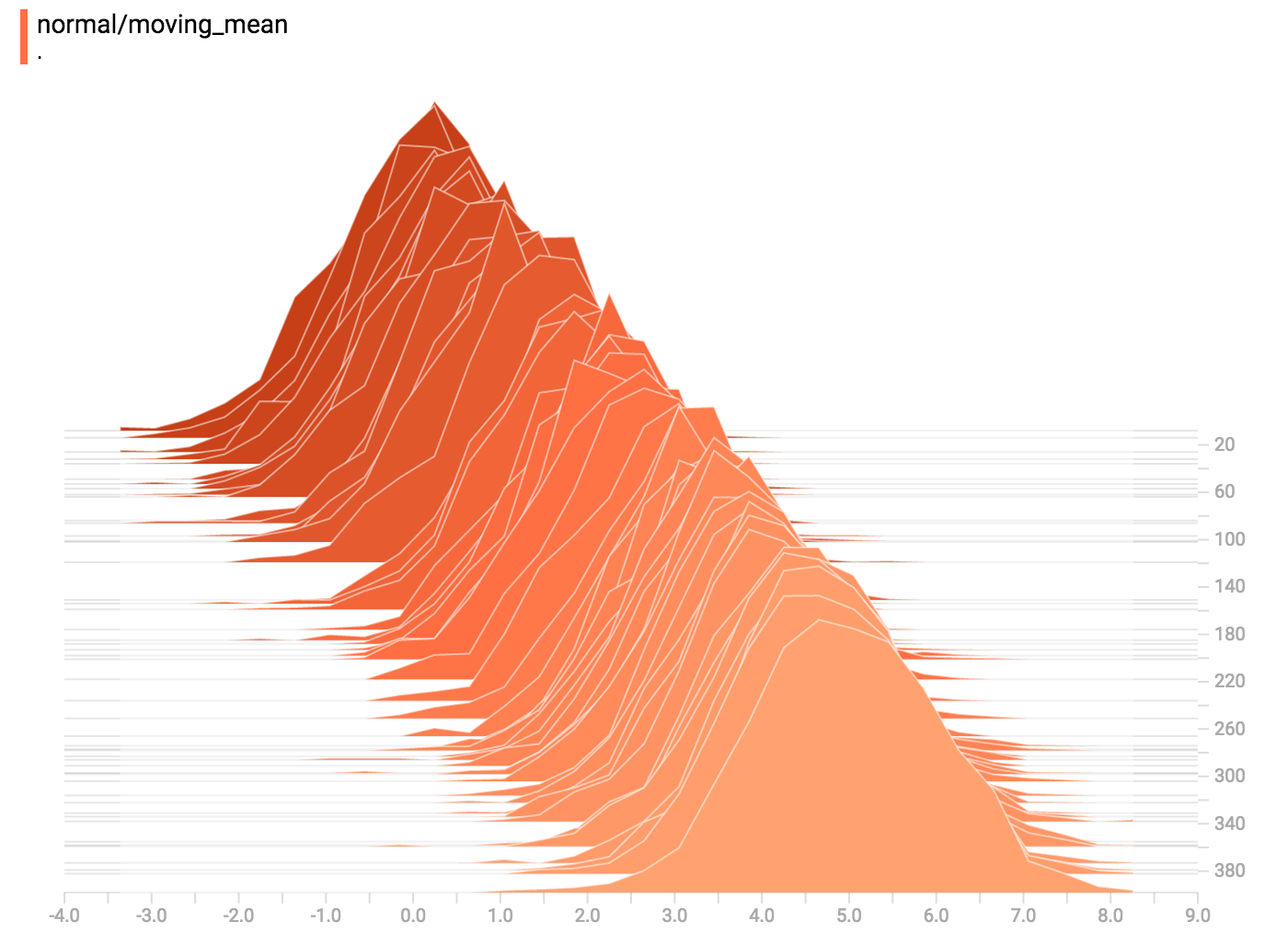`tf.summary.histogram` takes an arbitrarily sized and shaped Tensor, and compresses it into a histogram data structure consisting of many bins with widths and counts. For example, let's say we want to organize the numbers `[0.5, 1.1, 1.3, 2.2, 2.9, 2.99]` into bins. We could make three bins: * a bin containing everything from 0 to 1 (it would contain one element, 0.5), * a bin containing everything from 1-2 (it would contain two elements, 1.1 and 1.3), * a bin containing everything from 2-3 (it would contain three elements: 2.2, 2.9 and 2.99).

TensorFlow uses a similar approach to create bins, but unlike in our example, it doesn't create integer bins. For large, sparse datasets, that might result in many thousands of bins. Instead, the bins are exponentially distributed, with many bins close to 0 and comparatively few bins for very large numbers. However, visualizing exponentially-distributed bins is tricky; if height is used to encode count, then wider bins take more space, even if they have the same number of elements. Conversely, encoding count in the area makes height comparisons impossible. Instead, the histograms resample the data into uniform bins. This can lead to unfortunate artifacts in some cases.

Each slice in the histogram visualizer displays a single histogram. The slices are organized by step; older slices (e.g. step 0) are further "back" and darker, while newer slices (e.g. step 400) are close to the foreground, and lighter in color. The y-axis on the right shows the step number.

You can mouse over the histogram to see tooltips with some more detailed information. For example, in the following image we can see that the histogram at timestep 176 has a bin centered at 2.25 with 177 elements in that bin.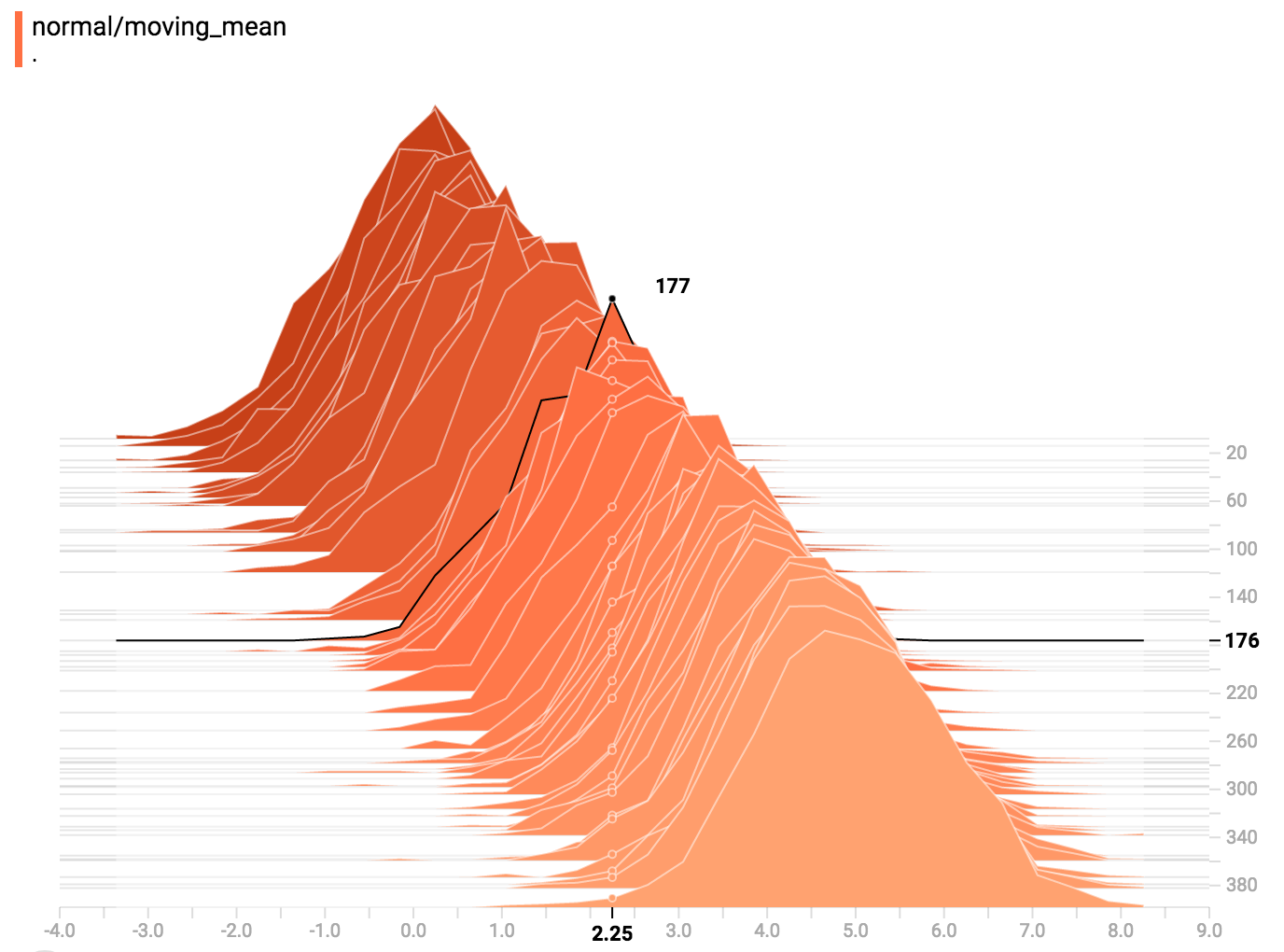Also, you may note that the histogram slices are not always evenly spaced in step count or time. This is because TensorBoard uses reservoir sampling to keep a subset of all the histograms, to save on memory. Reservoir sampling guarantees that every sample has an equal likelihood of being included, but because it is a randomized algorithm, the samples chosen don't occur at even steps.

## Overlay Mode

There is a control on the left of the dashboard that allows you to toggle the histogram mode from "offset" to "overlay":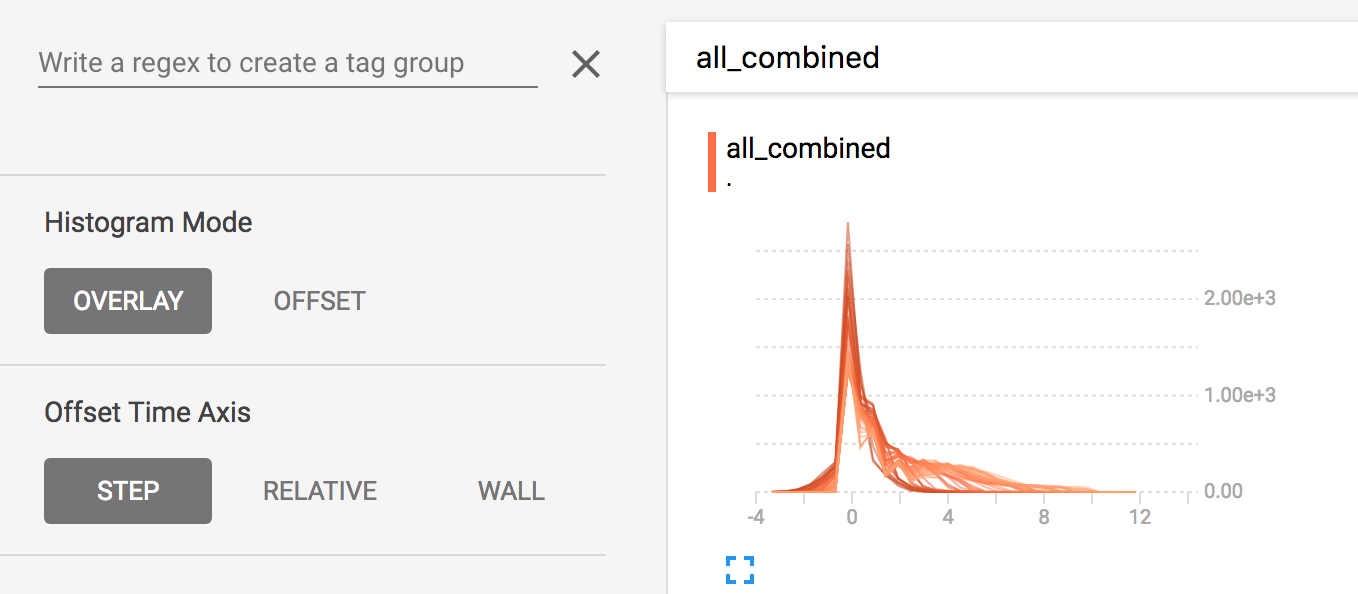In "offset" mode, the visualization rotates 45 degrees, so that the individual histogram slices are no longer spread out in time, but instead are all plotted on the same y-axis.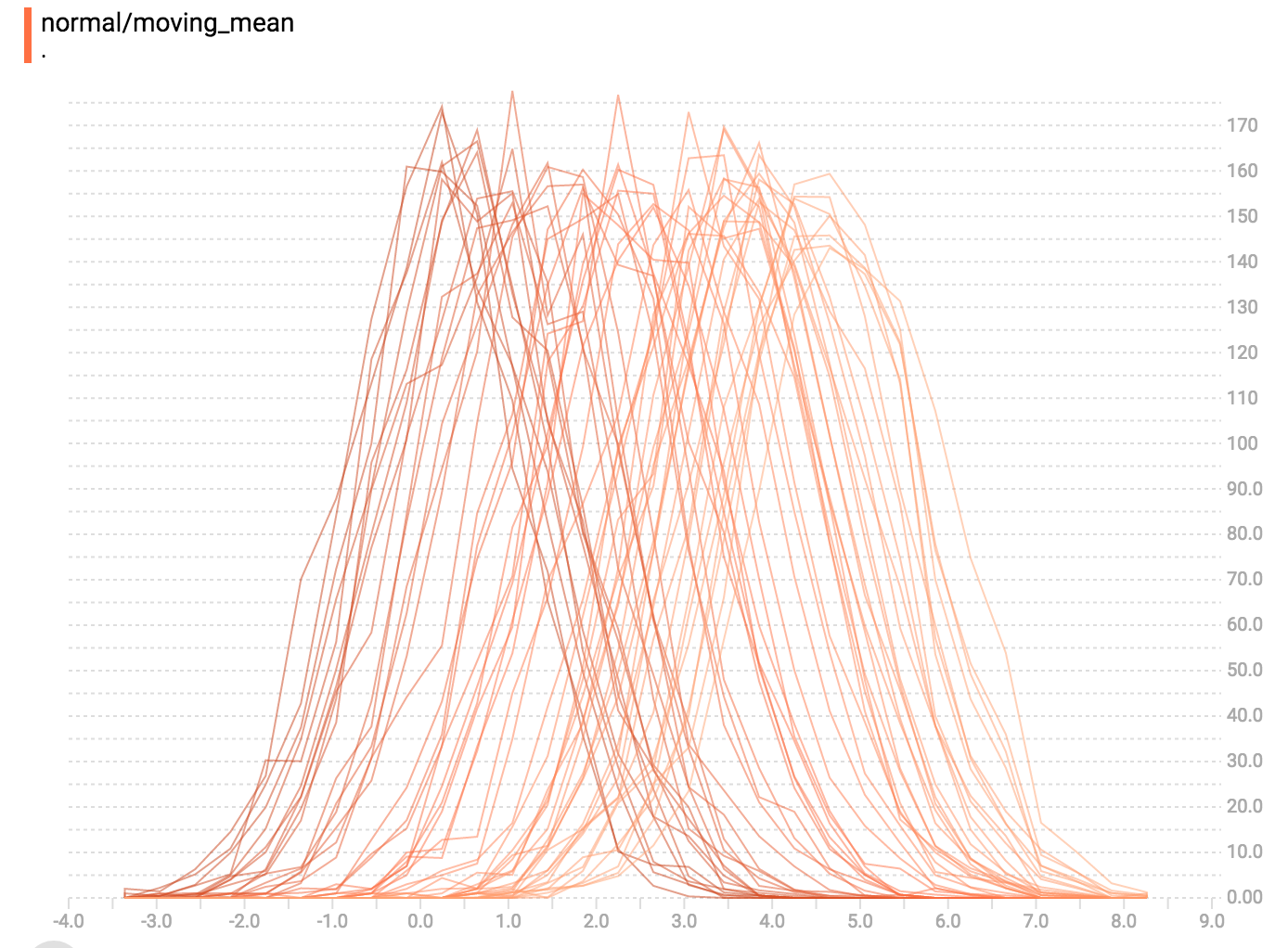Now, each slice is a separate line on the chart, and the y-axis shows the item count within each bucket. Darker lines are older, earlier steps, and lighter lines are more recent, later steps. Once again, you can mouse over the chart to see some additional information.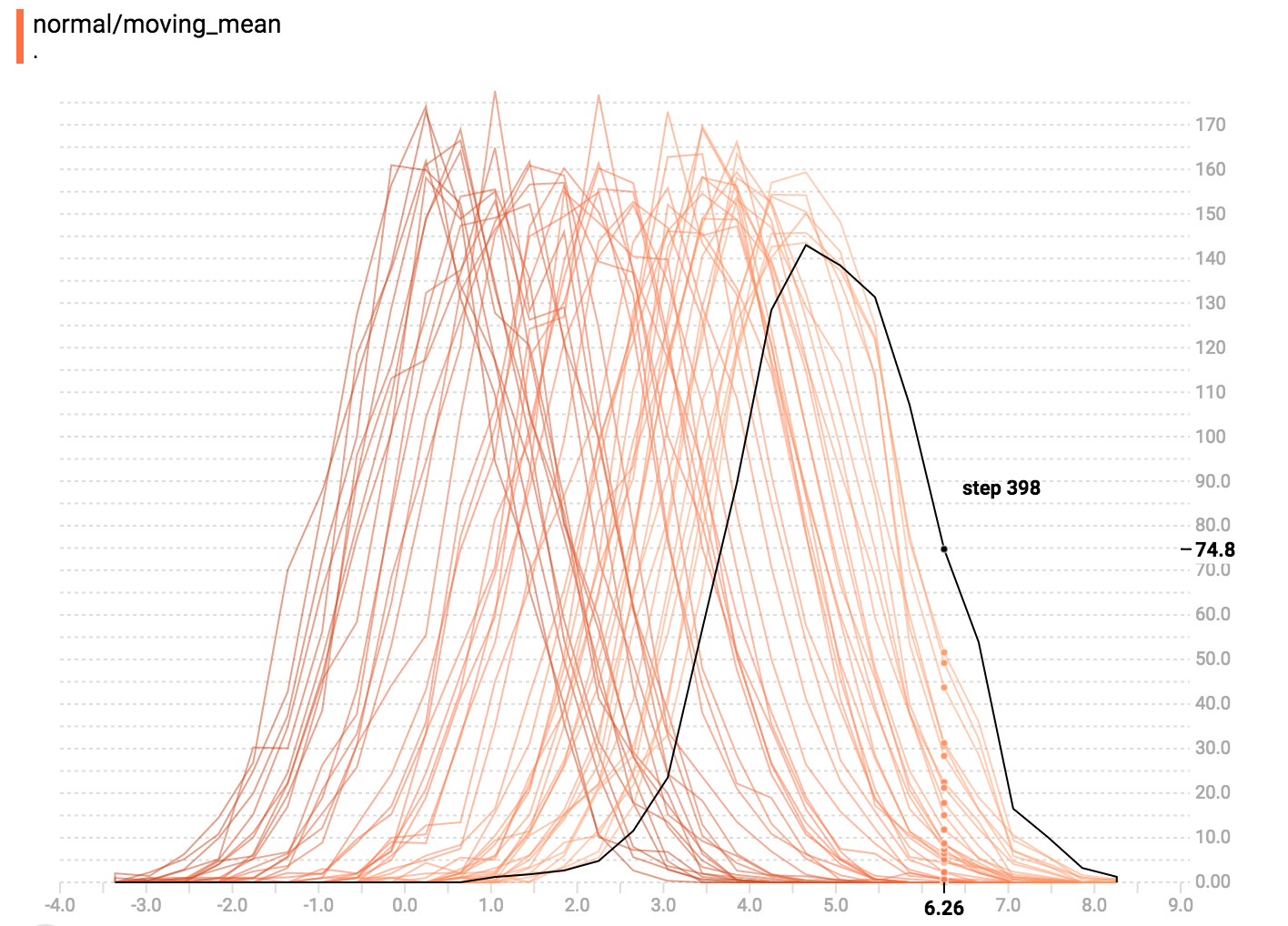In general, the overlay visualization is useful if you want to directly compare the counts of different histograms.

## Multimodal Distributions

The Histogram Dashboard is great for visualizing multimodal distributions. Let's construct a simple bimodal distribution by concatenating the outputs from two different normal distributions. The code will look like this:

``````import tensorflow as tf

k = tf.placeholder(tf.float32)

# Make a normal distribution, with a shifting mean
mean_moving_normal = tf.random_normal(shape=, mean=(5*k), stddev=1)
# Record that distribution into a histogram summary
tf.summary.histogram("normal/moving_mean", mean_moving_normal)

# Make a normal distribution with shrinking variance
variance_shrinking_normal = tf.random_normal(shape=, mean=0, stddev=1-(k))
# Record that distribution too
tf.summary.histogram("normal/shrinking_variance", variance_shrinking_normal)

# Let's combine both of those distributions into one dataset
normal_combined = tf.concat([mean_moving_normal, variance_shrinking_normal], 0)
# We add another histogram summary to record the combined distribution
tf.summary.histogram("normal/bimodal", normal_combined)

summaries = tf.summary.merge_all()

# Setup a session and summary writer
sess = tf.Session()
writer = tf.summary.FileWriter("/tmp/histogram_example")

# Setup a loop and write the summaries to disk
N = 400
for step in range(N):
k_val = step/float(N)
summ = sess.run(summaries, feed_dict={k: k_val})
``````

You already remember our "moving mean" normal distribution from the example above. Now we also have a "shrinking variance" distribution. Side-by-side, they look like this: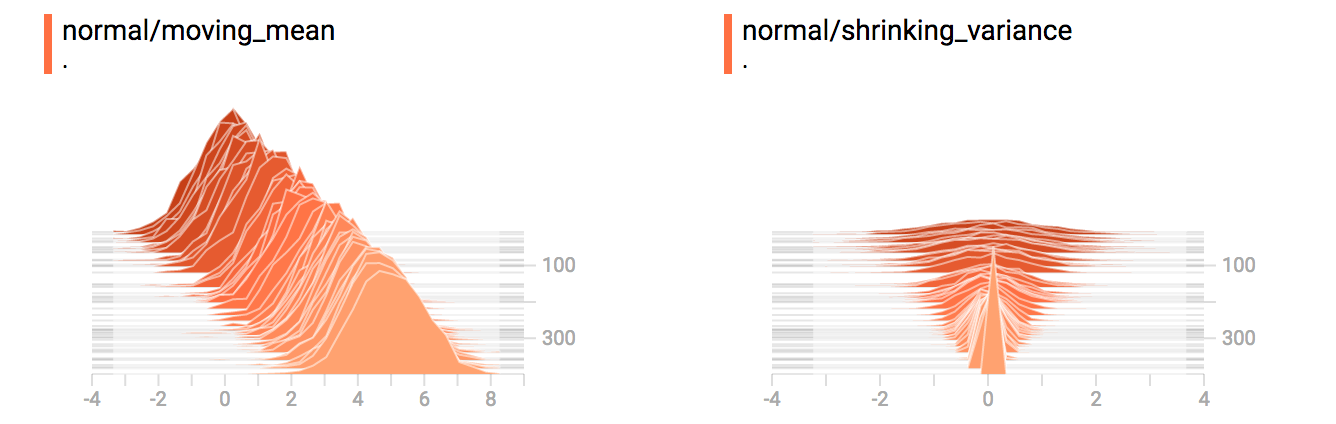When we concatenate them, we get a chart that clearly reveals the divergent, bimodal structure: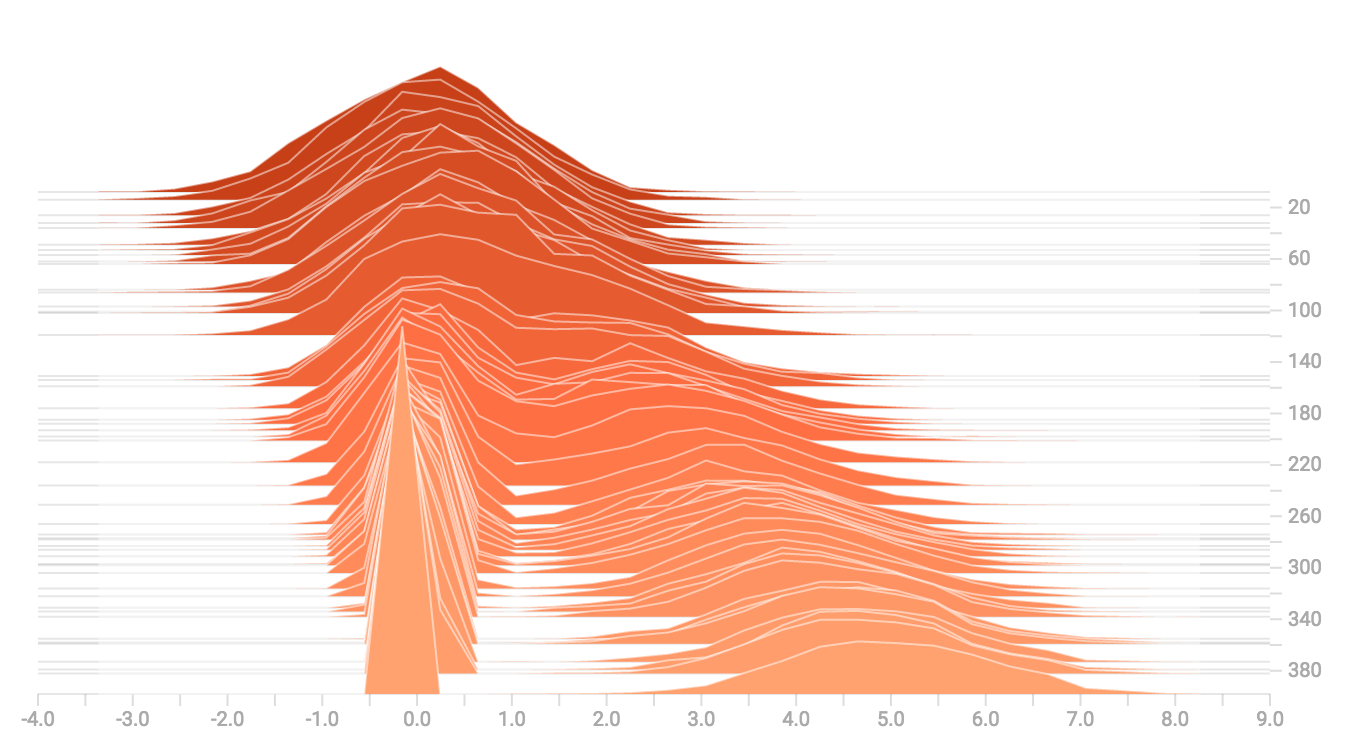## Some more distributions

Just for fun, let's generate and visualize a few more distributions, and then combine them all into one chart. Here's the code we'll use:

``````import tensorflow as tf

k = tf.placeholder(tf.float32)

# Make a normal distribution, with a shifting mean
mean_moving_normal = tf.random_normal(shape=, mean=(5*k), stddev=1)
# Record that distribution into a histogram summary
tf.summary.histogram("normal/moving_mean", mean_moving_normal)

# Make a normal distribution with shrinking variance
variance_shrinking_normal = tf.random_normal(shape=, mean=0, stddev=1-(k))
# Record that distribution too
tf.summary.histogram("normal/shrinking_variance", variance_shrinking_normal)

# Let's combine both of those distributions into one dataset
normal_combined = tf.concat([mean_moving_normal, variance_shrinking_normal], 0)
# We add another histogram summary to record the combined distribution
tf.summary.histogram("normal/bimodal", normal_combined)

gamma = tf.random_gamma(shape=, alpha=k)
tf.summary.histogram("gamma", gamma)

# And a poisson distribution
poisson = tf.random_poisson(shape=, lam=k)
tf.summary.histogram("poisson", poisson)

# And a uniform distribution
uniform = tf.random_uniform(shape=, maxval=k*10)
tf.summary.histogram("uniform", uniform)

# Finally, combine everything together!
all_distributions = [mean_moving_normal, variance_shrinking_normal,
gamma, poisson, uniform]
all_combined = tf.concat(all_distributions, 0)
tf.summary.histogram("all_combined", all_combined)

summaries = tf.summary.merge_all()

# Setup a session and summary writer
sess = tf.Session()
writer = tf.summary.FileWriter("/tmp/histogram_example")

# Setup a loop and write the summaries to disk
N = 400
for step in range(N):
k_val = step/float(N)
summ = sess.run(summaries, feed_dict={k: k_val})
``````

### Gamma Distribution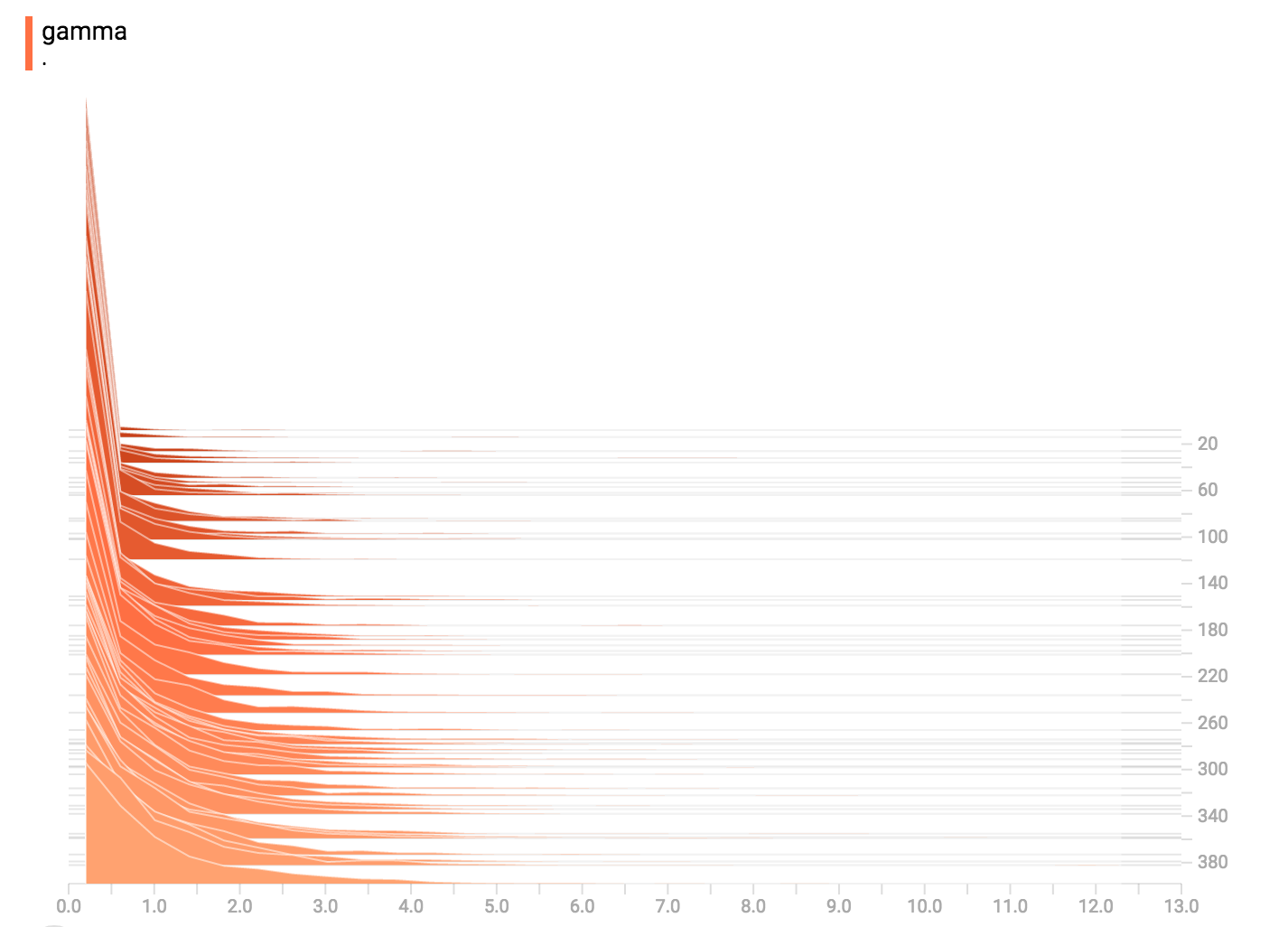### Uniform Distribution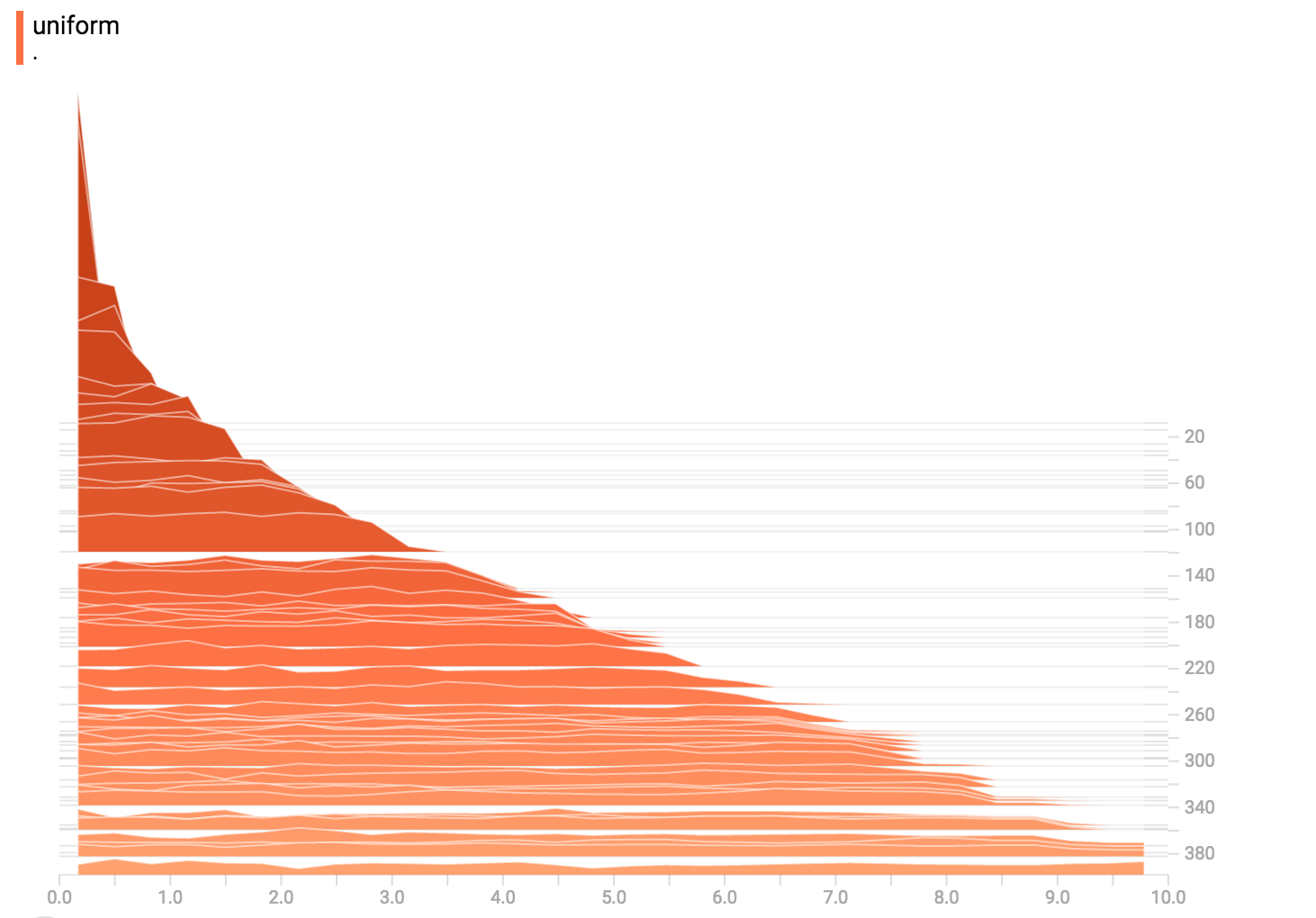### Poisson Distribution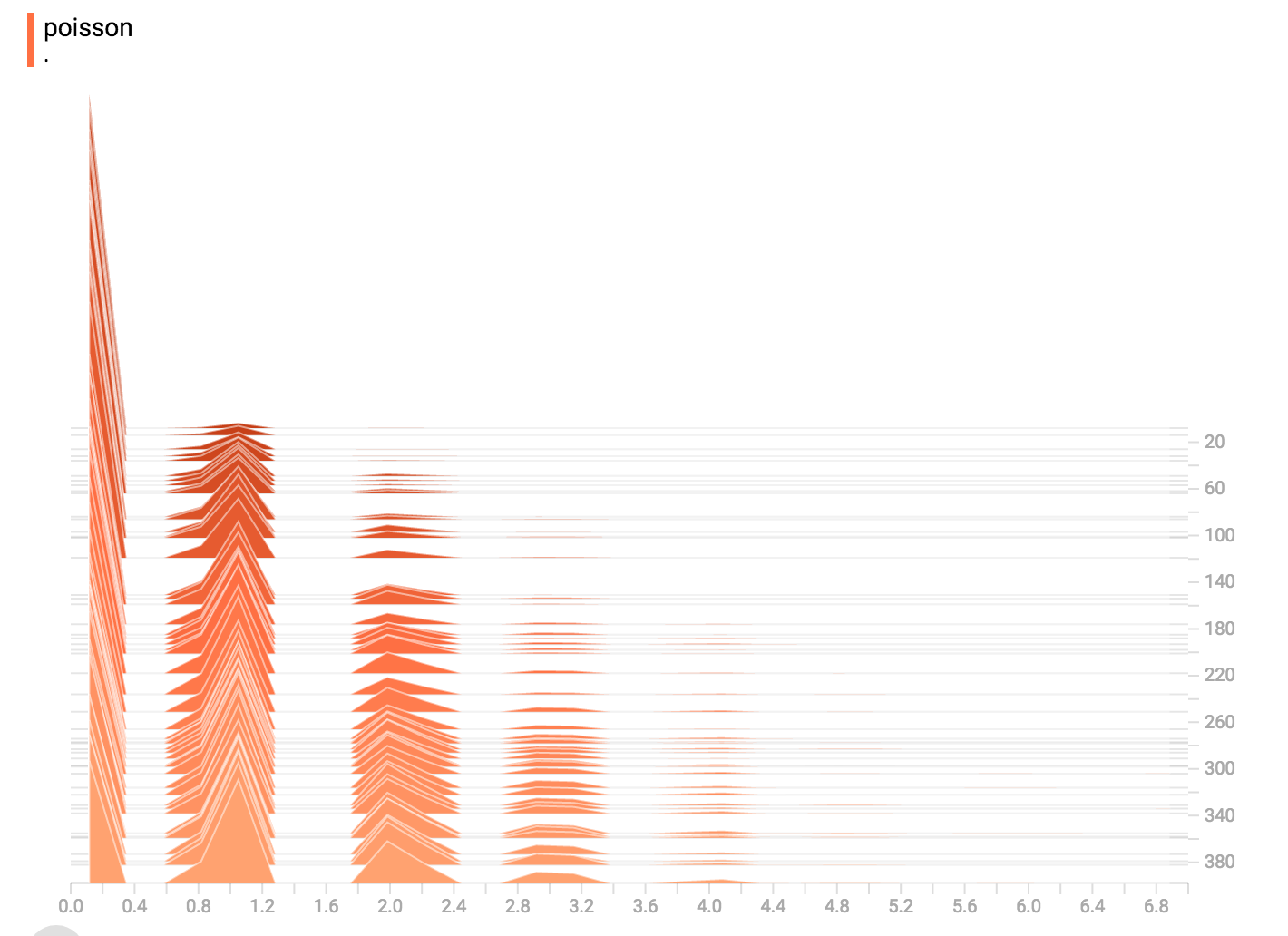The poisson distribution is defined over the integers. So, all of the values being generated are perfect integers. The histogram compression moves the data into floating-point bins, causing the visualization to show little bumps over the integer values rather than perfect spikes.

### All Together Now

Finally, we can concatenate all of the data into one funny-looking curve.